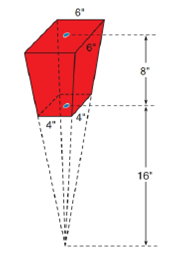Chapter 9.2, Problem 36E### Elementary Geometry for College St...

6th Edition
Daniel C. Alexander + 1 other
ISBN: 9781285195698

#### Solutions

Chapter
Section### Elementary Geometry for College St...

6th Edition
Daniel C. Alexander + 1 other
ISBN: 9781285195698
Textbook Problem
8 views

# A popcorn container at a movie theatre has the shape of a frustum of a pyramid (see Exercise 35). With dimensions as indicated, find the volume (capacity) of the container.To determine

To find:

The volume (capacity) of the container.

Explanation

Given:

The popcorn container at a movie theatre is in the shape of a frustum of a pyramid as shown below

Properties Used:

A pyramid is made by connecting a base to an apex. The base is flat with straight edges, no curves, hence, a polygon and all other faces are triangles.

A regular pyramid is a pyramid whose base is a regular polygon and whose lateral edges are all congruent.

A frustum of a pyramid is the portion of a pyramid that lies between its base and a parallel plane that intersects the pyramid.

The volume V of a pyramid having a base area B and an altitude of length h is given by V=13Bh.

According to the Pythagorean theorem, in a right-angled triangle

hypotenuse2=base2+perpendicular2.

In a regular pyramid, the lengths of the apothem a of the base, the altitude h, and the slant height l satisfy the Pythagorean Theorem; that is, l2=a2+h2.

Approach Used:

i) Calculate the volume of the bigger pyramid VB.

ii) Calculate the volume of the smaller pyramid VS.

iii) Subtract the two to get the volume of the frustum VF=VBVS.

Calculation:

Calculating the volume of the bigger pyramid

with base as a square of side x=6in and height as h=24in.

The area of the square base is

B=x2=62B=36in2

### Still sussing out bartleby?

Check out a sample textbook solution.

See a sample solution

#### The Solution to Your Study Problems

Bartleby provides explanations to thousands of textbook problems written by our experts, many with advanced degrees!

Get Started

#### Find more solutions based on key concepts